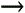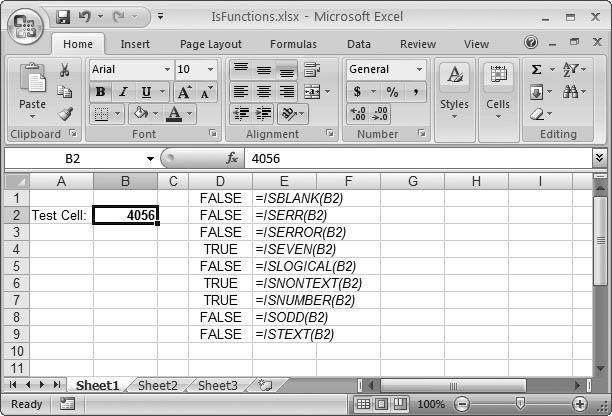Section 12.3. Information Functions

12.3. Information Functions

Excel features yet another group of functions closely related to the lookup and reference functions. Called information functions , they let you retrieve information about the type of content found in any particular cell you want to examine. You can find the full list of information functions in the Information group (choose FormulasFunction LibraryMore FunctionsInformation).

12.3.1. The "IS" Functions: Checking the Value Inside a Cell

The most important information functions are those that start with the word IS. These functions let you test whether a cell's blank, has numeric content, and so on.

The IS functions are Boolean functions, which means they give you a result of either TRUE or FALSE. On their own, the IS functions aren't too impressive. However, you can combine them with other conditional functions to make simple decisions. (The IF( ) function tests a condition, and then inserts one of two values based on whether the condition's true or false. For a refresher on the IF( ) function, see Section 8.1.6.)

You could use the IF( ) function in combination with the ISNUMBER( ) function to avoid performing a calculation if a cell doesn't contain numeric content. Here's the formula you'd use:

=IF(ISNUMBER(A1), 10/A1, NA())

In this example, if the ISNUMBER( ) test returns TRUE (that is, if A1 contains a number), then Excel uses the first argument. That means the program performs the calculation 10/A1 , and displays the result in the cell. If cell A1 doesn't contain numeric content, the ISNUMBER( ) function returns FALSE, and Excel uses the second argument. In this case, Excel executes the NA( ) function, which displays the error code #N/A. Figure 12-11 shows several IS functions at work.

Here's another example that displays an error message when an error exists in a cell:

=IF(ISERROR(D10), "The calculation could not be completed due to an error.", "")

You could insert this formula in cell E10, so it appears right next to the cell that may or may not have an error (cell D10). If an error doesn't exist, E10 stays blank. If an error does occur, then E10 offers a little bit of extra information.

Excel 2007 adds a handy shortcut for error checking with a new function named IFERROR( ). IFERROR( ) checks the indicated cell for an error, and then displays one of two values depending on the result. The previous example, which uses both IF( ) and ISERROR( ), can be written in a slightly more compact way using IFERROR( ), like so:

=IFERROR(D10, "The calculation could not be completed due to an error.", "")Figure 12-11. This worksheet shows the result of a series of tests on a single cell, B2. On their own, these TRUE and FALSE results may not impress you, but they represent building blocks you can use to create powerful conditional expressions.

IFERROR( ) may not save much typing, but it does make for more readable formulas.

Table 12-2 lists the IS functions. Usually, you'll use the IS functions with a literal value or a cell reference, although you can also use a range. When you use a range, the value is true only if the condition is satisfied in all the cells in the range. That means that =ISBLANK(A1:A10) returns TRUE only if all 10 cells in the range are empty.

Table 12-2. The IS Functions

Function

Returns TRUE If

ISBLANK( )

The cell is empty, meaning it doesn't contain text, numbers , or any other content.

ISERR( )

The cell contains an error other than #N/A.

ISERROR( )

The cell contains any error, including #N/A.

ISEVEN( )

The value is an even number.

ISLOGICAL( )

The value is a Boolean (TRUE or FALSE) value.

ISNA( )

The cell contains the #N/A error.

ISNONTEXT( )

The value isn't text; it could be blank, a number, or a Boolean value.

ISNUMBER( )

The value is a number, and not blank.

ISODD( )

The value is an odd number.

ISREF( )

The value is a cell reference. Thus, ISREF(A1) is true, but ISREF("A1") is not.

ISTEXT( )

The value is text, and not blank.

12.3.2. TYPE( ) and ERROR.TYPE( ): Finding a Value's Data Type or Error Type

Both the TYPE( ) and ERROR.TYPE( ) functions examine a cell and return a number that describes its content. You can use these functions to build conditional formulas (Section 8.1.6).

The TYPE( ) function returns a number that represents the type of data in a cell. Possible numbers include:

• 1 (Number)

• 2 (Text)

• 4 (Logical Value)

• 16 (Error)

• 64 (Range)

The ERROR.TYPE( ) returns a number that represents the type of error that's occurred in a cell. If the cell doesn't contain an error, the ERROR.TYPE( ) function returns #N/A. Possible numbers include:

• 1 (#NULL!)

• 2 (#DIV/0!)

• 3 (#VALUE!)

• 4 (#REF!)

• 5 (# NAME ?)

• 6 (#NUM!)

• 7 (#N/A)

To learn how you to use these kinds of functions to build conditional logic, see Chapter 13.

12.3.3. INFO( ) and CELL( ): Gathering Info About Your Computer and Your Worksheet's Cells

INFO( ) and CELL( ) are two of the strangest information functions out there. While they're useful for stumping co-workers with Excel trivia, they don't solve many practical problems.

The INFO( ) function provides information related to the computer and operating system that's running Excel. To kick it into gear, you specify a single text argument, which tells Excel what information to retrieve. If you specify "numfile," Excel returns the number of all the worksheets in all the workbook files that are currently open :

=INFO("numfile")

Note: As you can see here, you need to enclose the argument value in quotation marks.

Table 12-3 lists all the possible arguments you can use with the INFO( ) function. Possible, of course, doesn't mean any of these make it into heavy rotation.

Table 12-3. Argument Values for the INFO( ) Function

Text

Gives You

directory

The current directory path. This path is usually the last directory you browsed to in the Open or Save dialog boxes, which means that it's not necessarily the path where Excel's stored the current spreadsheet.

memavail

The current amount of your computer's available memory, in bytes.

memused

The current amount of your computer's used memory, in bytes.

numfile

The number of all the worksheets in all the workbook files that are currently open.

origin

The reference, as text, of the cell in the top-left corner of the currently visible area. The cell reference is prepended with the fixed text \$A. This trick is useful for backward compatibility with ancient versions of the Lotus 1-2-3 spreadsheet program.

osversion

The version of your computer's operating system.

recalc

The current recalculation mode, which determines when formula results are refreshed. Chapter 13 (Section 13.4) describes the different recalculation modes and how to set them.

release

The version of Excel.

system

The type of operating system"pcdos" for a PC, or "mac" for a Macintosh.

totmem

The total memory of the computer, including memory that's currently in use.

The CELL( ) function performs a similar trick, except that it returns information about a cell. It requires two arguments: a string indicating the type of information you want, and a reference pointing to the cell you want to examine. Here's an example that retrieves the number format of the cell A2:

=CELL("format", A2)

If you omit the cell reference, the CELL( ) function operates on the cell where the formula exists. The CELL( ) function is useful primarily for maintaining compatibility with other software. People occasionally use it in add-ins or macros that automate tasks in a worksheet, although most of its features are duplicated by other, more straightforward information and reference functions like ROW( ), COLUMN(), INDIRECT( ), and ADDRESS( ). One interesting piece of information that you can retrieve from CELL( ), but not from these other functions, is the file name (including the full file path) of the current spreadsheet:

=CELL("filename")

For a full list of values you can use with the CELL( ) function, refer to the Excel help referenceor just start typing. As soon as you add the CELL( ) function to a formula and press the opening parenthesis, Excel pops up a list of possible values that you can choose from.

Tip: The CELL( ) and INFO( ) functions don't refresh automatically. If you need to recalculate these formulas, then press F9 to update the worksheet.Excel 2007[c] The Missing Manual
ISBN: 596527594
EAN: N/A
Year: 2007
Pages: 173